### Moments of Inertia of area: Composite areas

In the context of calculating the moment of inertia, a composite area is an area consisting of several non-overlapping (disjoint) sub-areas. The boundaries specifying the sub-areas can be explicitly declared by the geometry or arbitrarily chosen. Considering an area as a composite area is to simplify the calculation of the moment of inertia of the whole area. having simple shapes with already known or given formulations of moments of inertia

Consider an area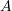consisting of (or partitioned into) n disjoint sub-areas denoted as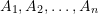(Fig. 10.12).

Then by the definition (Eq. 10.1), the moment of inertial ofabout an axis m can be written as,

(10.10)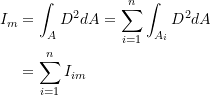where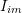denotes the moment of inertia of the i-th area about the m axis. This results expresses that the moment of inertia of an area about an axis (m) is the sum of the moments of inertia of its (disjoint) sub-areas about the same common axis (m).

The same result also holds for the polar moments of inertia of an area and its sub-areas; meaning that,

(10.11)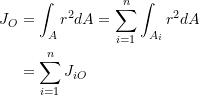where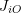denotes the polar moment of inertia of the i-th area about the (common) point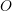.

Remark: When we sum the values of the moments of inertia (of sub-areas), the values have to be calculated about a common axis. A hint for problem solving is to use the parallel axis theorem regarding the common axis.

#### EXAMPLE 10.4.1

Determine the moments of inertia of the depicted area about the x and y axes shown. Hint: Use the formulas shown in Fig. 10.11 and the parallel axis theorem.

#### SOLUTION

Partition the area into simple disjoint shapes (areas) with known (or can be easily found) centroids. The location of centroids is needed as per the parallel axis theorem. One possible choice of sub-area is shown below. The centroids are determined using the information from Fig. 9.11.

Part 1: moment of inertia about the x axis.

To calculate the moment of inertia, of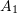about, we can use the parallel axes theorem. To this end, we need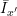being the moment of inertia ofabout the centroidal axis,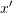, parallel to. Theaxis and its distance to theaxis are shown in the figure below.

Letting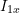be the moment of inertia ofabout the, we can write,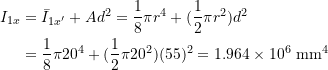Similarly, area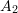and the axis crossing its centroid and parallel to theaxis are considered and shown as below.

Therefore,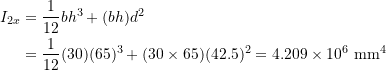For area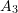, we have the following configuration.

Therefore,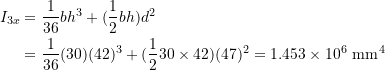Now, adding the moment of inertia of each part (about the common axis x) will give the moment of inertia of the whole area about the x axis as,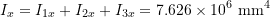The above calculations can be summarized in a table as follows.

Part 2: moment of inertia about the y axis.

To calculate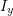of areaas shown below, we should note that the formula (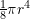) in Fig. 10.11 already expresses the moment of inertia of a semi-circular area about the axis along its base.

Therefore,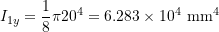For area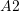as shown, we should use the parallel axes theorem.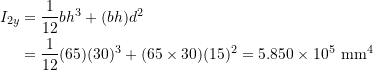And for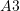,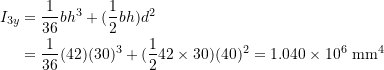Consequently,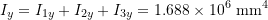The above calculations can be summarized in a table as follows.

In many cases, the sub-areas are explicitly pronounced by the geometry of the area. The following example shows an instance.

#### EXAMPLE 10.4.2

For the displayed I-shaped cross-section of a beam, find the moments of inertia about the horizontal and vertical axes crossing the centroid of the area.

#### SOLUTION

Partition the area into disjoint sub-area and determine the centroid of the area.

The area can be partitioned into the sub-areas shown in the figure below. The centroid of each sub-area is determined and a Cartesian coordinate system is set to facilitate the calculation.

Although we can calculate it, we conclude, by the symmetry, that,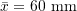And,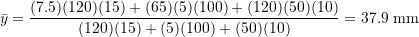Follow the steps as shown in the previous example for each sub-area. The formula for calculating the moment of inertia of a rectangular area about a centroidal axis is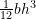as shown in Fig. 10.11.

Calculating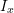: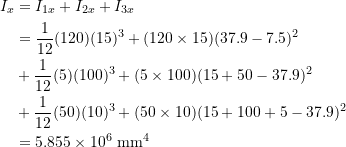The calculations can also be tabulated as,

Calculating: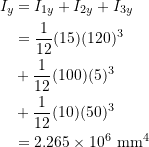which can be tabulated as,

The following interactive example demonstrates a beam cross section, its centroid and its moments of inertia about the axes (horizontal and vertical) crossing the centroid of the area. You can change the dimensions of each sub-area and also set the positions of the flanges (top parts) by horizontally dragging them. The purpose of this example is to let you observe the variations of the location of the centroid and the moments of inertia as you change the shape of the area. Nevertheless, it is recommended that you try to calculate some cases yourselves and check your answers. The unit of length, in the example, is arbitrary; you can assume milliliter.

##### Moment of inertia of an area with empty parts

As discussed in Section 9.3.5, an empty part (hollow region) of an area is treated as a negative area in the formulations. Consequently, an empty area has a negative value of moment of inertia.

#### EXAMPLE 10.4.3

Determineandof the beam cross sectional area about the x and y axes as shown.

#### SOLUTION

As shown in the figure below, the area can be considered as the blue rectangular area () minus the green rectangular area ().

By symmetry, we recognize that the x and y axes are crossing the centroid of the rectangular areas. Therefore (using the formula in Fig. 10.11),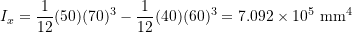The calculations can be organized in a table as,

And,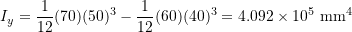which can be tabulated as,

### Video

a https://www.engineeringintro.com/mechanics-of-structures/moment-of-inertia/moment-of-inertia-of-hollow-section/